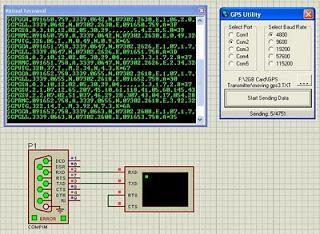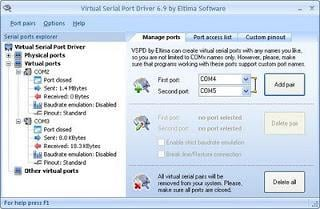Virtual GPS Engine for simulation in Proteus VSM.

This post describes a method for reading a text file into Proteus VSM. The text file is comprised of unprocessed GPS data that was created by traveling in a vehicle while carrying a laptop with the GPS engine connected. The data is to be used for simulation and post-processing purposes.

Raw and unparsed GPS data was sent to a text file using hyper-terminal in the laptop that was connected to the GPS engine while being transported in a moving vehicle. The data is required to be processed by Proteus Simulation software so would have to be read into Proteus VSM from the text file. From there the text file will be used to develop an algorithm for parsing and post-processing.

These components are required to perform this procedure:

Eltima’s Virtual Serial Port Driver Software (VSPD) which will be used to create virtual serial ports.

Proteus VSM Software

Visual Basic 6 code developed to read data from the text file of GPS data and transfer it to the Proteus COMPIM model.

Eltima Virtual Serial Port Driver and the Proteus COMPIM model are used to send the GPS data saved in the text file through a Visual Basic 6 program and then to the COMPIM model in the Proteus simulation.

Figure1 below shows the circuit schematic drawn in Proteus VSM:Figure 1: Circuit Schematic

The results of a test run of this simulation are displayed in Figure 2 below:Figure 2: Test run of the Simulation

Figure 3 is a screenshot taken from the VSPD interface that shows the creation of the virtual serial port connection.Figure 3: Eltima Software Interface

This simulation is designed to develop code that can be used in real hardware controllers to parse and extract information from raw GPS data. We accomplish this by reading the text file into the Proteus VSM simulation software. The data is then sent to a serial port in a simulation of a micro-controller enabling us to test the code’s real-world behavior.

The Visual Basic 6 project is shown in a screenshot in Figure 4 below:Figure 4: Screen Shot of VB project

Figure 4 provides a snapshot of the VB code for the project. The code can be linked to at:

```        ```Public Function ReadLine(fname As String, LineNumber As Long) _
As String

Dim oFSO As New FileSystemObject
Dim oFSTR As Scripting.TextStream
Dim lCtr As Long

If oFSO.FileExists(fname) Then
Set oFSTR = oFSO.OpenTextFile(fname)
Do While Not oFSTR.AtEndOfStream
lCtr = lCtr + 1
If lCtr = LineNumber Then
Exit Do
End If
oFSTR.SkipLine
Loop
oFSTR.Close
Set oFSTR = Nothing

End If
End Function

Public Function SaveTextToFile(FileFullPath As String, _
sText As String, Optional Overwrite As Boolean = False) As _
Boolean

On Error GoTo ErrorHandler
Dim iFileNumber As Integer
iFileNumber = FreeFile

If Overwrite Then
Open FileFullPath For Output As #iFileNumber
Else
Open FileFullPath For Append As #iFileNumber
End If

Print #iFileNumber, sText
SaveTextToFile = True

ErrorHandler:
Close #iFileNumber
End Function

VB6 Code 02 (File named : TextFiles.cls) :

Option Explicit

Private m_sFilePath As String
Private m_lSize As Long
Private strLines() As String
Private m_lines As Long
Public completeFile As String

m_lines = 0
Dim fso As New Scripting.FileSystemObject
Dim ts As Scripting.TextStream
Dim str As String

'// Store size
m_lSize = fso.GetFile(m_sFilePath).Size

Dim i As Long
i = 0
ReDim Preserve strLines(0 To 800000) As String

'// Get number of lines
Set ts = fso.OpenTextFile(m_sFilePath, ForReading, False)

While Not ts.AtEndOfStream
i = i + 1
strLines(i - 1) = str
m_lines = i
'completeFile = completeFile & str
Wend
completeFile = Join(strLines, vbCrLf)
'// Close file
ts.Close

'// Release objects
Set ts = Nothing
Set fso = Nothing

End Sub
Public Property Get ReadLines(n As Long, m As Long) As String
Dim buf As String
buf = ""
Dim i As Long
For i = n To m
If i >= n And i <= m Then
buf = buf & strLines(i - 1) & vbCrLf
DoEvents
End If
If i > m Then
Exit Property
End If

Next
End Property

Public Property Get ReadLine(ByVal n As Long) As String
If n <= 0 Then n = 1
If n >= m_lines Then n = m_lines
End Property

Public Property Let TextFilePath(ByVal pVal As String)

m_sFilePath = pVal

End Property

Public Property Get FileSize() As Long

FileSize = m_lSize
End Property

Public Property Get NoOfLines() As Long
NoOfLines = m_lines
End Property

Public Sub WriteNewLine(ByVal str As String)
m_lines = m_lines + 1
ReDim Preserve strLines(0 To m_lines) As String
strLines(m_lines - 1) = str
WriteFile

End Sub
Private Sub WriteFile()
Dim i As Long
i = 0
Dim fso As New Scripting.FileSystemObject
Dim ts As Scripting.TextStream

Set ts = fso.OpenTextFile(m_sFilePath, ForWriting, False)

For i = 0 To m_lines - 1
ts.WriteLine (strLines(i))
Next
ts.Close

'// Release objects
Set ts = Nothing
Set fso = Nothing

End Sub

Private Sub WriteFileAtPosi(ByVal str As String, ByVal posi As Long)
Dim i As Long
i = 0
Dim fso As New Scripting.FileSystemObject
Dim ts As Scripting.TextStream

Set ts = fso.OpenTextFile(m_sFilePath, ForWriting, False)

For i = 0 To m_lines - 1
If (i = posi) Then
ts.WriteLine (str)
End If
ts.WriteLine (strLines(i))
Next
ts.Close

'// Release objects
Set ts = Nothing
Set fso = Nothing

End Sub

Public Sub InsertNewLine(ByVal str As String, ByVal posi As Long)
WriteFileAtPosi str, posi
End Sub

Private Sub ReplaceFileAtPosi(ByVal str As String, ByVal posi As Integer)
Dim i As Integer
i = 0
Dim fso As New Scripting.FileSystemObject
Dim ts As Scripting.TextStream

Set ts = fso.OpenTextFile(m_sFilePath, ForWriting, False)

For i = 0 To m_lines - 1
If (i = posi - 1) Then
ts.WriteLine (str)
i = i + 1
End If
ts.WriteLine (strLines(i))

Next
ts.Close

'// Release objects
Set ts = Nothing
Set fso = Nothing

End Sub

Public Sub ReplaceLine(ByVal str As String, ByVal LineNum As Integer)
ReplaceFileAtPosi str, LineNum
End Sub

Public Sub DeleteLines()
Dim i As Long
i = 0
Dim fso As New Scripting.FileSystemObject
Dim ts As Scripting.TextStream

Set ts = fso.OpenTextFile(m_sFilePath, ForWriting, False)

For i = 5000 To m_lines - 1
ts.WriteLine (strLines(i))
Next
ts.Close

'// Release objects
Set ts = Nothing
Set fso = Nothing